# GENERAL TREES BINARY TREES 1 TREES Previous data

• Slides: 27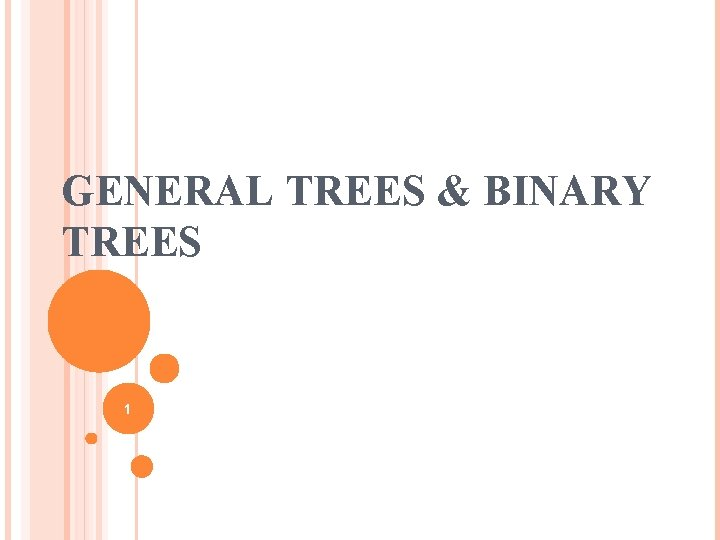GENERAL TREES & BINARY TREES 1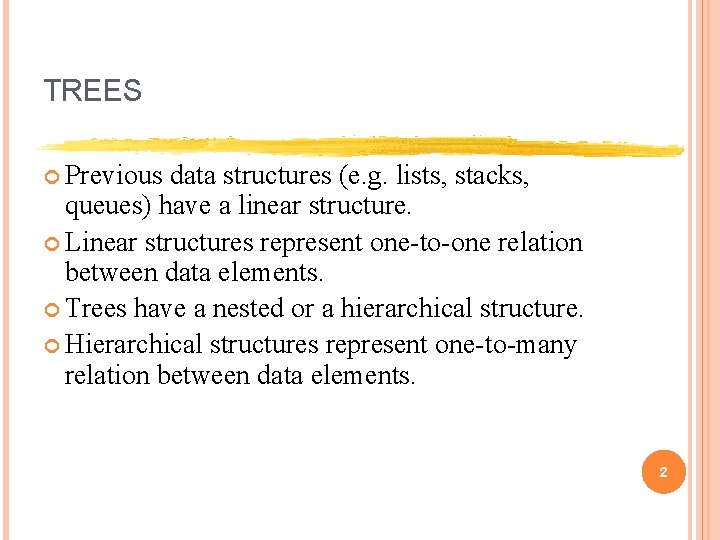TREES Previous data structures (e. g. lists, stacks, queues) have a linear structure. Linear structures represent one-to-one relation between data elements. Trees have a nested or a hierarchical structure. Hierarchical structures represent one-to-many relation between data elements. 2TREES Examples of situations were one-to-many relations exist… these can be represented as trees. Relation between a parent and his children. Relation between a person and books he owns. Relation between a basketball team and the players on the team. Card catalog in a library. 3TREES: SOME TERMINOLOGY A tree is represented as a set of nodes connected by edges. Root A Tree Nodes Edges Leafs 4TREES: COMPARISON WITH LISTS A List Unique first element. Unique last element. Each element, other than the first and the last, has a unique predecessor and a unique successor. A Tree Unique first node called root. Each node has successors, called its children. Each node has one predecessor, called parent. Leafs have no children. Root has no parent. 5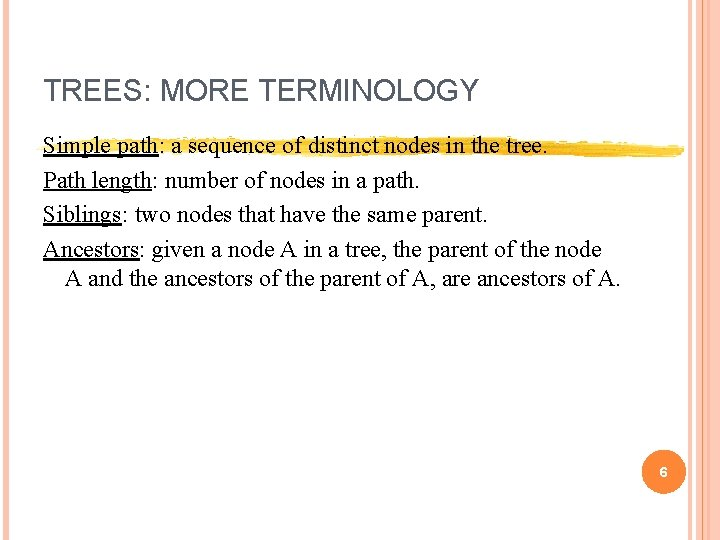TREES: MORE TERMINOLOGY Simple path: a sequence of distinct nodes in the tree. Path length: number of nodes in a path. Siblings: two nodes that have the same parent. Ancestors: given a node A in a tree, the parent of the node A and the ancestors of the parent of A, are ancestors of A. 6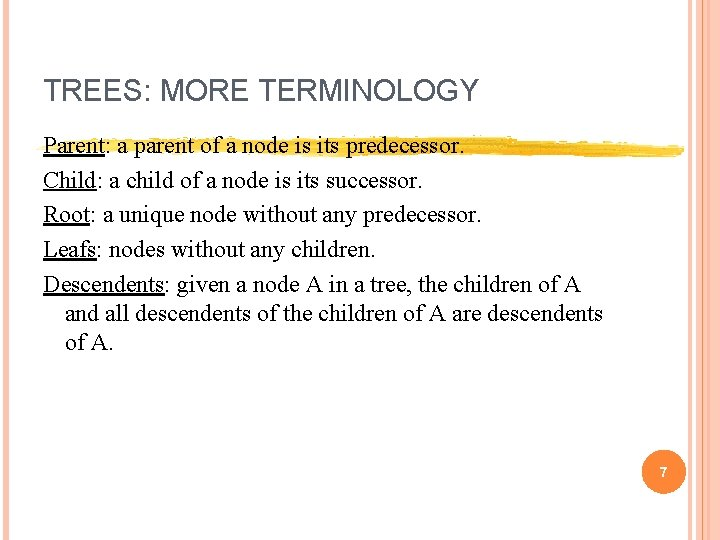TREES: MORE TERMINOLOGY Parent: a parent of a node is its predecessor. Child: a child of a node is its successor. Root: a unique node without any predecessor. Leafs: nodes without any children. Descendents: given a node A in a tree, the children of A and all descendents of the children of A are descendents of A. 7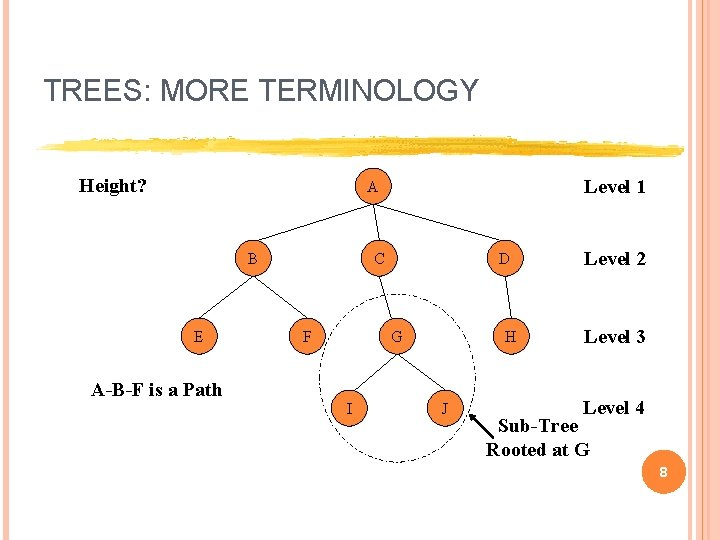TREES: MORE TERMINOLOGY Height? Level 1 A B E A-B-F is a Path C F D G I H J Level 2 Level 3 Level 4 Sub-Tree Rooted at G 8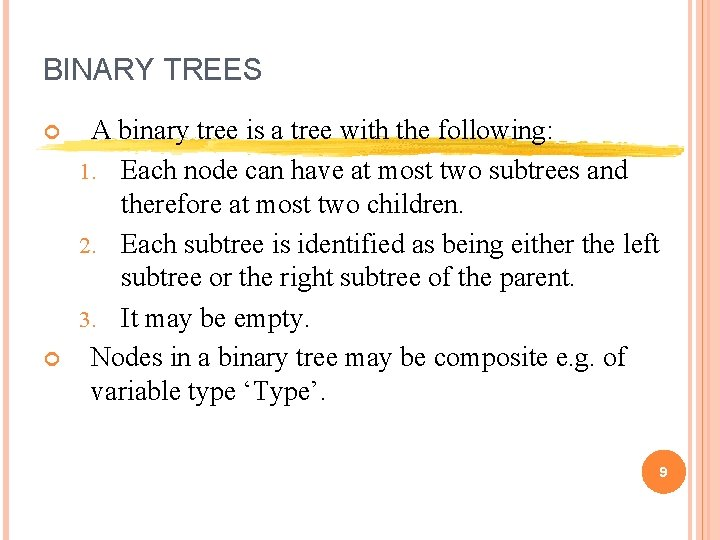BINARY TREES A binary tree is a tree with the following: 1. Each node can have at most two subtrees and therefore at most two children. 2. Each subtree is identified as being either the left subtree or the right subtree of the parent. 3. It may be empty. Nodes in a binary tree may be composite e. g. of variable type ‘Type’. 9BINARY TREES + * [(a + b) * c] + 7 * + + 7 c a + b c 7 (a + b) * (c + 7) a b 10ADT BINARY TREE Elements: The elements are nodes, each node contains the following data type: Type and has Left. Child and Right. Child references. Structure: hierarchical structure; each node can have two children: left or right child; there is a root node and a current node. Domain: the number of nodes in a binary tree is bounded; domain contains empty tree, trees with one element, two elements, … 11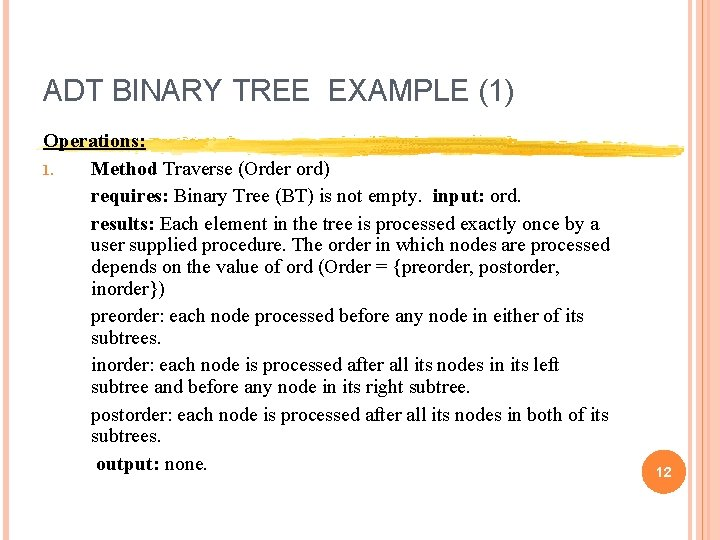ADT BINARY TREE EXAMPLE (1) Operations: 1. Method Traverse (Order ord) requires: Binary Tree (BT) is not empty. input: ord. results: Each element in the tree is processed exactly once by a user supplied procedure. The order in which nodes are processed depends on the value of ord (Order = {preorder, postorder, inorder}) preorder: each node processed before any node in either of its subtrees. inorder: each node is processed after all its nodes in its left subtree and before any node in its right subtree. postorder: each node is processed after all its nodes in both of its subtrees. output: none. 12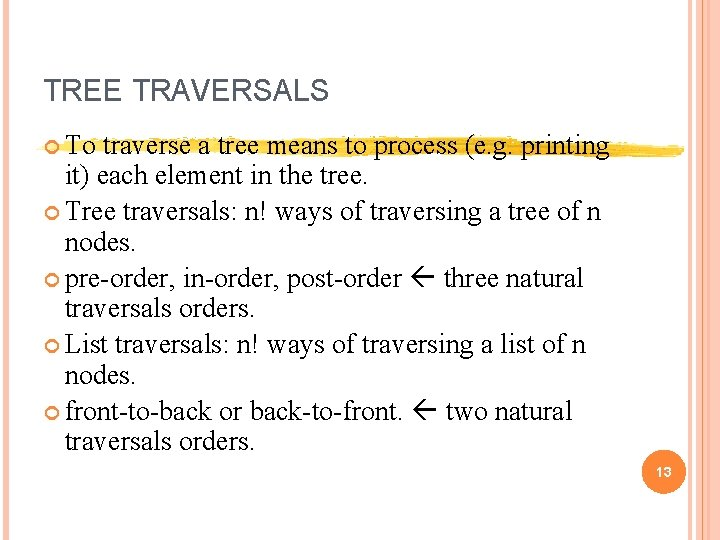TREE TRAVERSALS To traverse a tree means to process (e. g. printing it) each element in the tree. Tree traversals: n! ways of traversing a tree of n nodes. pre-order, in-order, post-order three natural traversals orders. List traversals: n! ways of traversing a list of n nodes. front-to-back or back-to-front. two natural traversals orders. 13ADT BINARY TREE Operations: 2. Method Insert (Type e, Relative rel, boolean inserted) (Relative = {leftchild, rightchild, root, parent}) requires: (1) Full () is false and (2) either (a) rel = root and Empty() is true or (b) rel <> root and rel <> parent and Empty( ) is false. input: e, rel. results: if case (1) rel = left. Child, current node has a left child, or (2) rel = right. Child, current node has a right child, then inserted is false. Else a node containing e is added as rel of the current node in the tree and becomes the current node and inserted is true. output: inserted. 14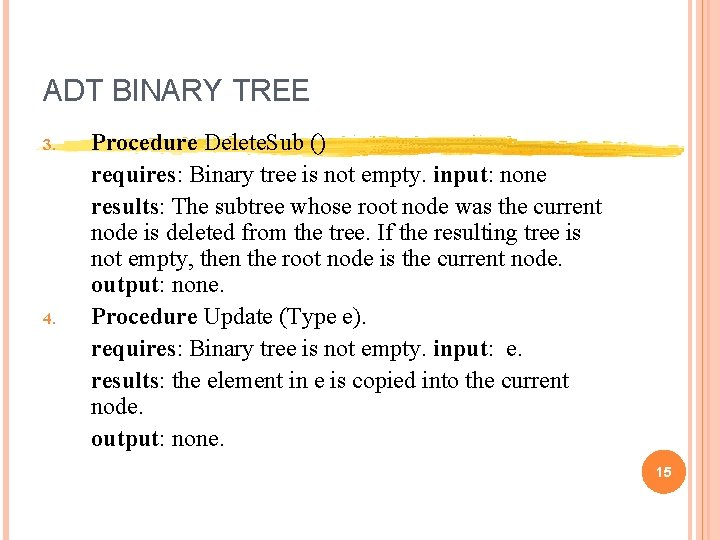ADT BINARY TREE 3. 4. Procedure Delete. Sub () requires: Binary tree is not empty. input: none results: The subtree whose root node was the current node is deleted from the tree. If the resulting tree is not empty, then the root node is the current node. output: none. Procedure Update (Type e). requires: Binary tree is not empty. input: e. results: the element in e is copied into the current node. output: none. 15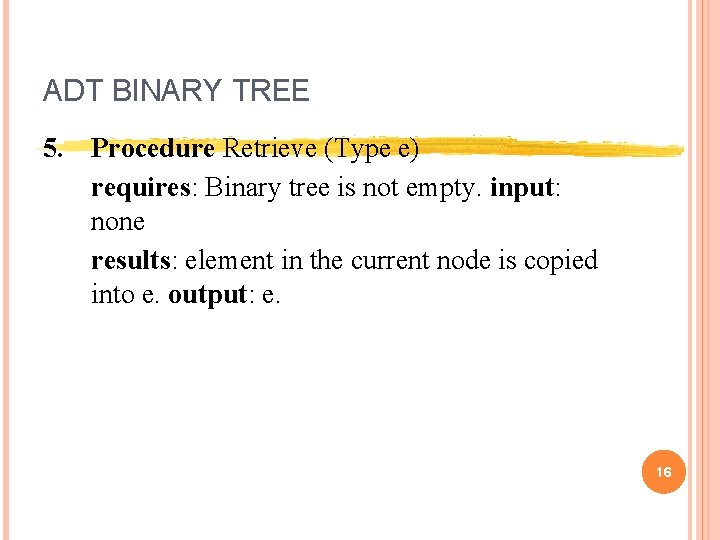ADT BINARY TREE 5. Procedure Retrieve (Type e) requires: Binary tree is not empty. input: none results: element in the current node is copied into e. output: e. 16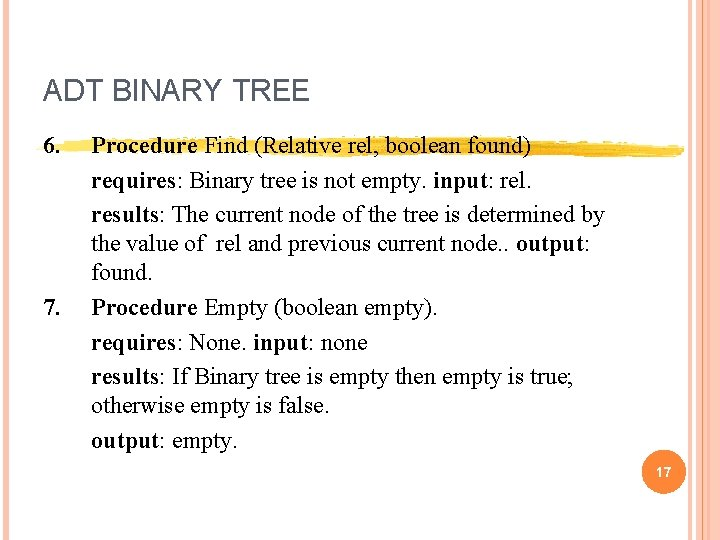ADT BINARY TREE 6. 7. Procedure Find (Relative rel, boolean found) requires: Binary tree is not empty. input: rel. results: The current node of the tree is determined by the value of rel and previous current node. . output: found. Procedure Empty (boolean empty). requires: None. input: none results: If Binary tree is empty then empty is true; otherwise empty is false. output: empty. 17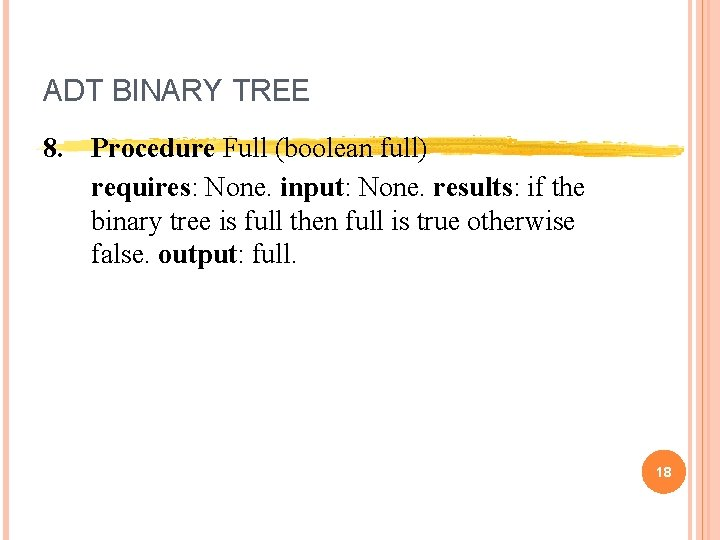ADT BINARY TREE 8. Procedure Full (boolean full) requires: None. input: None. results: if the binary tree is full then full is true otherwise false. output: full. 18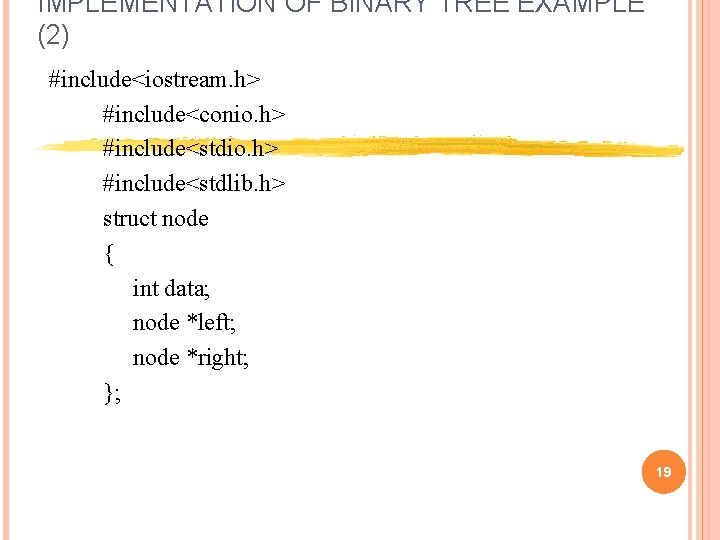IMPLEMENTATION OF BINARY TREE EXAMPLE (2) #include<iostream. h> #include<conio. h> #include<stdlib. h> struct node { int data; node *left; node *right; }; 19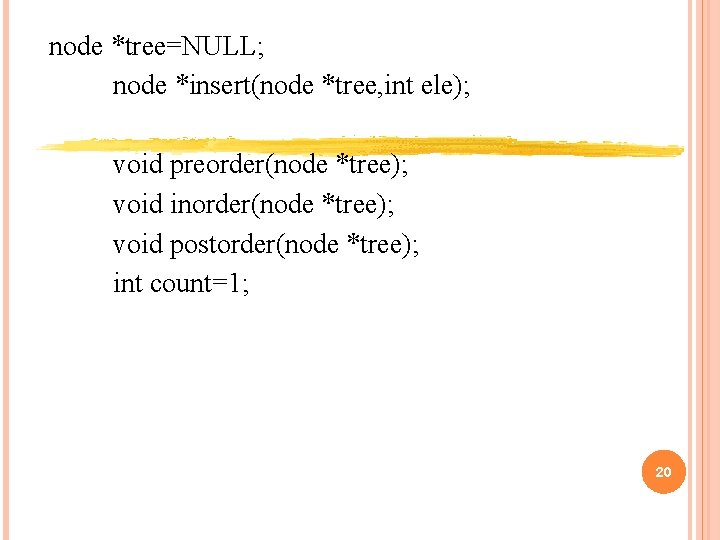node *tree=NULL; node *insert(node *tree, int ele); void preorder(node *tree); void inorder(node *tree); void postorder(node *tree); int count=1; 20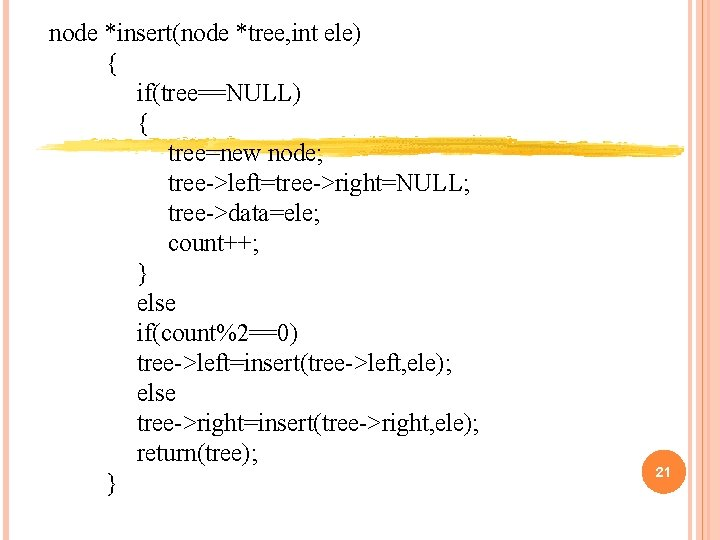node *insert(node *tree, int ele) { if(tree==NULL) { tree=new node; tree->left=tree->right=NULL; tree->data=ele; count++; } else if(count%2==0) tree->left=insert(tree->left, ele); else tree->right=insert(tree->right, ele); return(tree); } 21void preorder(node *tree) { if(tree!=NULL) { cout<<tree->data; preorder(tree->left); preorder(tree->right); getch(); } } 22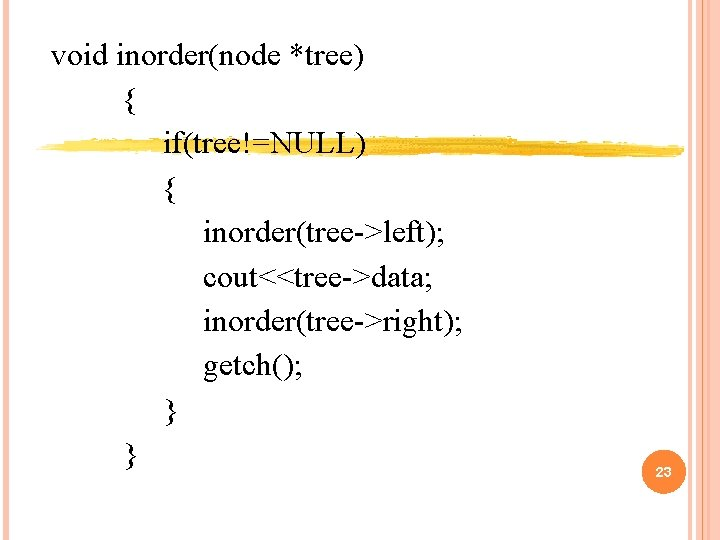void inorder(node *tree) { if(tree!=NULL) { inorder(tree->left); cout<<tree->data; inorder(tree->right); getch(); } } 23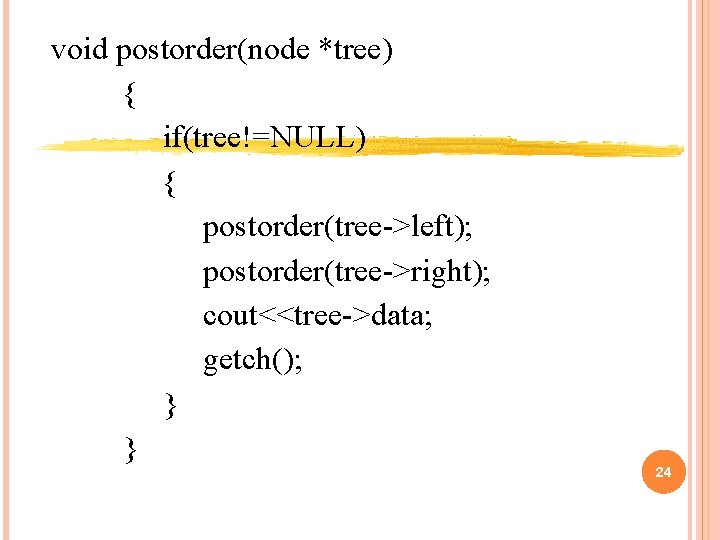void postorder(node *tree) { if(tree!=NULL) { postorder(tree->left); postorder(tree->right); cout<<tree->data; getch(); } } 24void main() { clrscr(); int ch, ele; do { clrscr(); cout<<"ntaa 1 ----INSERT A NODE IN A BINARY TREE. aa"; cout<<"ntaa 2 ----PRE-ORDER TRAVERSAL. aa"; cout<<"ntaa 3 ----IN-ORDER TRAVERSAL. aa"; cout<<"ntaa 4 ----POST-ORDER TRAVERSAL. aa"; cout<<"ntaa 5 ----EXIT. aa"; cout<<"ntaa. ENTER CHOICE: : aa"; cin>>ch; 25switch(ch) { case 1: cout<<"ntaa. ENTER THE ELEMENT: : aa"; cin>>ele; tree=insert(tree, ele); break; case 2: cout<<"ntaa****PRE-ORDER TRAVERSAL OF A TREE****aa"; preorder(tree); break; case 3: cout<<"ntaa****IN-ORDER TRAVERSAL OF A TREE****aa"; inorder(tree); break; 26case 4: cout<<"ntaa****POST-ORDER TRAVERSAL OF A TREE****aa"; postorder(tree); break; case 5: exit(0); } }while(ch!=5); } // end of main 27